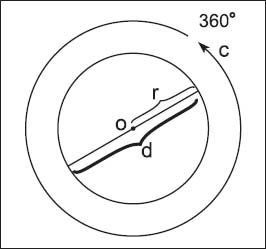NextPrevious

# What is a circle?

A circle is one of the most fundamental shapes in geometry—and one shape we commonly see every day. For mathematicians, a circle is defined as a set of points on a plane at a certain distance from a center point. In reality, a circle is a polygon with an infinite number of sides.

The distance of a line segment from the center to the points on the circle is called the radius (or a line segment whose endpoints are on any point on the circle and its center). A line segment that travels from one endpoint on the circle, through the radius (center of the circle), and to another endpoint directly opposite is called the diameter; two times the radius of a circle is the diameter. The outer perimeter of the circle is called the circumference. The chord of a circle is a line segment whose two endpoints are on the circle. Concentric circles are two or more circles that lie in the same plane and have the same center, but with different radii. Circles with the same radius are called congruent circles. (For more about circle measurements, see elsewhere in this chapter.)The parts of a circle include the center (o), radius (r), diameter (d), and circumference (c).

Close

This is a web preview of the "The Handy Math Answer Book" app. Many features only work on your mobile device. If you like what you see, we hope you will consider buying. Get the App